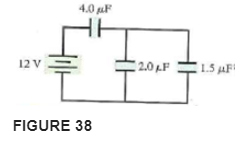# Problem: For the circuit of Figure 38, a. What is the equivalent capacitance?b. How much charge flows through the battery as the capacitors are being charged?

###### FREE Expert Solution

Equivalent capacitance for two capacitors in series:

$\overline{){{\mathbf{C}}}_{\mathbf{e}\mathbf{q}}{\mathbf{=}}\frac{{\mathbf{C}}_{\mathbf{1}}{\mathbf{C}}_{\mathbf{2}}}{{\mathbf{C}}_{\mathbf{1}}\mathbf{+}{\mathbf{C}}_{\mathbf{2}}}}$

For capacitors in parallel, the equivalent capacitance is:

$\overline{){{\mathbf{C}}}_{\mathbf{e}\mathbf{q}}{\mathbf{=}}{{\mathbf{C}}}_{{\mathbf{1}}}{\mathbf{+}}{{\mathbf{C}}}_{{\mathbf{2}}}{\mathbf{+}}{\mathbf{.}}{\mathbf{.}}{\mathbf{.}}{\mathbf{+}}{{\mathbf{C}}}_{{\mathbf{n}}}}$

(a)

The 2.0 μF and the 1.5 μF capacitors are in parallel:

Ceq = C1 + C2 = 2.0 + 1.5 = 3.5 μF

80% (1 ratings)###### Problem Details

For the circuit of Figure 38,

a. What is the equivalent capacitance?

b. How much charge flows through the battery as the capacitors are being charged?